### RANDBETWEEN Function Examples in Excel, VBA, & Google Sheets

This Tutorial demonstrates how to use the Excel RANDBETWEEN Function in Excel to calculate a random number between two numbers.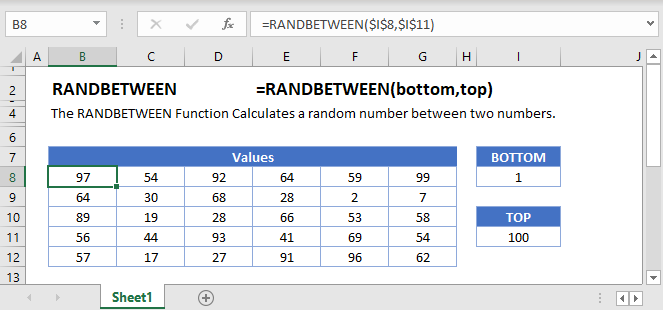## RANDBETWEEN Function Overview

The RANDBETWEEN Function Calculates a random number between two numbers.

To use the RANDBETWEEN Excel Worksheet Function, select a cell and type: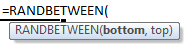(Notice how the formula inputs appear)

## RANDBETWEEN function Syntax and inputs:

`=RANDBETWEEN(bottom,top)`

bottom – The smallest integer that randbetween can return.

top – The largest integer that randbetween can return.

AutoMacro - VBA Code Generator

## How to use the RANDBETWEEN Function in Excel:

The RANDBETWEEN function generates numbers (whole numbers) between numbers you specify.

`=RANDBETWEEN(B3,C3)`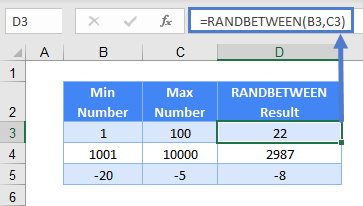Note: RANDARRAY is a new function in Excel 2019 onwards and replaces the RANDBETWEEN function. But if you don’t have the latest version or need to send the file out for people who don’t have it yet, you still can use the RANDBETWEEN function for backwards compatibility.

Besides generating random numbers you specify, RANDARRAY allows you to choose how many rows and columns to place the random numbers, and even choose if you want whole numbers or decimals.

If your Excel version is Excel 2003 and below, you can use RAND as RANDBETWEEN is only available from 2007 and above. Instead of allowing you to choose the minimum and maximum number, it randomly generates decimals between 0 and 1.

`=RAND()`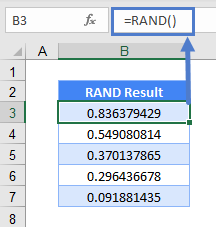## Generate Decimals within a Range

As mentioned, RANDARRAY and RAND do this easily. With RANDBETWEEN, you need to do it like this:

`=RANDBETWEEN(\$B\$3*10,\$B\$6*10)/10`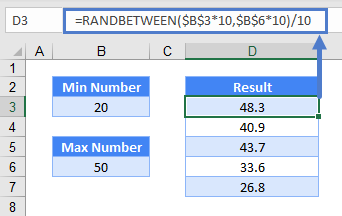You need to first decide how many decimal places you want. In the above eg, it’s one decimal place. And you need to divide a whole number by 10 to get one decimal place. Hence, the formula is basically

`=RANDBETWEEN(Minimum Number x 10, Maximum Number x 10) / 10.`

## Generate Random Dates or/and Time

If you know how dates and time are stored in Excel, the logic is going to similar to the one above because they are stored as numbers. Just:

`=RANDBETWEEN(\$B\$3,\$B\$6)`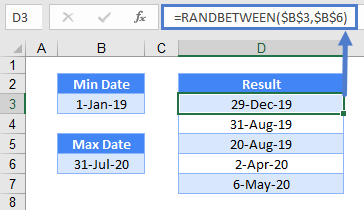Time is similar, except that it’s decimal instead of a whole number or integer. There are 24 hours a day, so multiply the minimum and maximum numbers by 24 and divide that random number by 24 to get a random hour.

`=RANDBETWEEN(\$B\$3*24,\$B\$6*24)/24`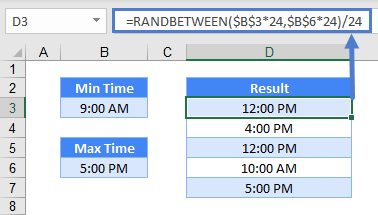If you want random minutes as well, use 1440 (24 hours x 60 minutes) instead of 24.

`=RANDBETWEEN(\$B\$3*1440,\$B\$6*1440)/1440`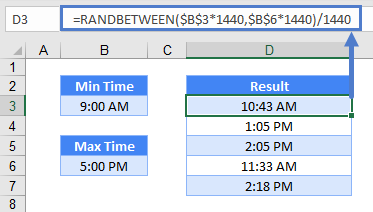To have them both together, simply add them together:

`=RANDBETWEEN(\$B\$3,\$B\$6)+RANDBETWEEN(\$C\$3*1440,\$C\$6*1440)/1440`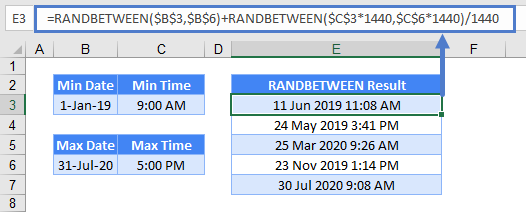# Retrieve a Random Field

If you simply need to randomize whole numbers, RANDBETWEEN is perfect by itself. Most of the time though, we want to retrieve a random field. To grab a random employee in B3:B7 for instance, you can use a RANDBETWEEN function to grab a row number, and INDEX to grab the particular value of the row:

`=INDEX(B3:B7,RANDBETWEEN(1,5))`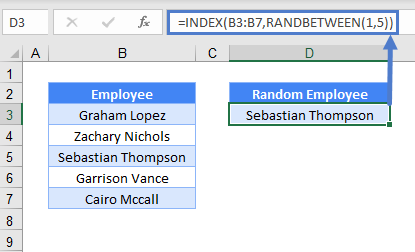# Retrieve a Random Field without Duplicates

To ensure there are no duplicates, you can use this array formula.

```{=INDEX(\$B\$3:\$B\$7,LARGE(IF(ISNA(MATCH(\$B\$3:\$B\$7,D\$2:D2,0)),ROW(\$B\$3:\$B\$7),0),
RANDBETWEEN(1,5-ROWS(D\$3:D3)+1))-ROW(\$B\$3)+1)}```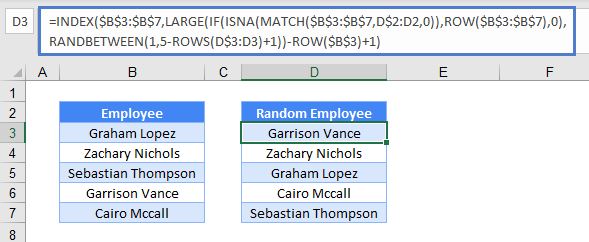In the above eg, RANDBETWEEN first randomizes between 1 and (5 – ROWS(D\$3:D3) + 1). Essentially, it’s 5 – 1 + 1 and still 5. As the formula is copied down, it randomizes between 5 and 4 (5 – 2 + 1), and slowly to just between 1 and 1.

This part of randomizing is to get the nth largest number. For the first row in cell D3, it’s fine to grab any number from 1 to 5 since there are no duplicates yet.

For the second row in cell D4, you only want it to randomize among 4 numbers since one is already taken. And the IF + ISNA + MATCH + ROW helps to return a row number only if there B3:B7 is not a match for cell D3. In the eg, Garrison Vance in D3 is a match in B3:B7. Hence, it only returns the row number for the rest of the names.

Don’t press Enter, but CTRL + SHIFT + ENTER because this is an array formula. You should see curly brackets surrounding it when done right. If you’re using Excel 2019 or Office 365, you can enter the formula normally

<<<Note to Steve: steve edit text – intro is confusing between rand vs randbetween>>>>

# RANDBETWEEN Function in Google Sheets

The RANDBETWEEN function works the same in Google Sheets.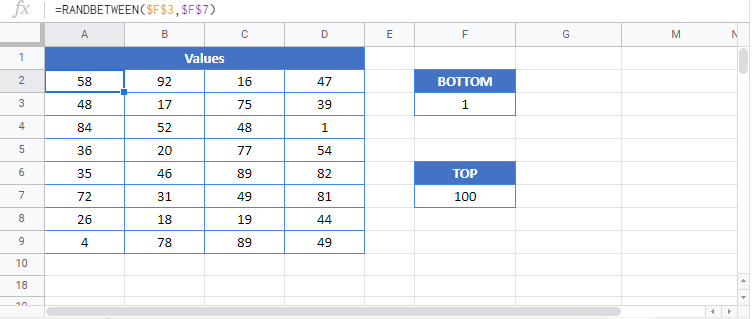## Additional Notes

Use the RANDBETWEEN Function to calculate a random integer between two numbers.
`=rand()`

## RANDBETWEEN Examples in VBAYou can also use the RANDBETWEEN function in VBA. Type:` Application.Worksheetfunction.Randbetween(bottom,top)`
For the function arguments (bottom, etc.), you can either enter them directly into the function, or define variables to use instead.

Executing the following VBA statements

```Range("C2") = Application.WorksheetFunction.RandBetween(Range("A2"), Range("B2"))
Range("C3") = Application.WorksheetFunction.RandBetween(Range("A3"), Range("B3"))
Range("C4") = Application.WorksheetFunction.RandBetween(Range("A4"), Range("B4"))```

will produce the following results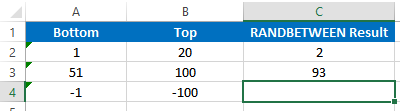The third statement will raise an error, as RANDBETWEEN will not accept negative numbers as parameters: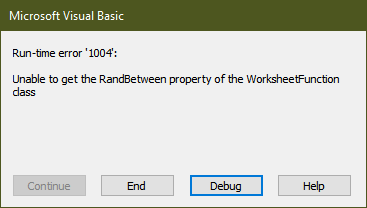Return to the List of all Functions in Excel

## Excel Practice Worksheet

Practice Excel functions and formulas with our 100% free practice worksheets!

• Automatically Graded Exercises
• Learn Excel, Inside Excel!